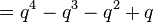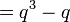# Endomorphism structure of general linear group of degree two over a finite field

This article gives specific information, namely, endomorphism structure, about a family of groups, namely: general linear group of degree two. This article restricts attention to the case where the underlying ring is a finite field.
View endomorphism structure of group families | View other specific information about general linear group of degree two | View other specific information about group families for rings of the type finite field

This article describes the endomorphism structure of the general linear group of degree two over a finite field.

## Particular cases

The information below is for the group$GL(2,q)$ where$q$ is a prime power and$p$ is the underlying prime (i.e., the field characteristic). Here,$\varphi$ here denotes the Euler totient function. Sort by$p$ to separate the characteristic two cases from the other cases.

Group$p$$q$$r = \log_pq$ Order of the group$= q^4 - q^3 - q^2 + q$ Automorphism group Order of the automorphism group$= r(q^3 - q)\varphi(2(q - 1))$
Order of inner automorphism group ($= q^3 - q$) Order of radial automorphism group ($= \varphi(2(q-1))$) Order of Galois group ($= r$) Endomorphism structure page
symmetric group:S3 2 2 1 6 symmetric group:S3 6 6 1 1 endomorphism structure of symmetric group:S3
general linear group:GL(2,3) 3 3 1 48 direct product of S4 and Z2 48 24 2 1 endomorphism structure of general linear group:GL(2,3)
direct product of A5 and Z3 2 4 2 180 direct product of A5 and V4 240 60 2 2 --
general linear group:GL(2,5) 5 5 1 480 480 120 4 1 endomorphism structure of general linear group:GL(2,5)
general linear group:GL(2,7) 7 7 1 2016 1344 336 4 1 endomorphism structure of general linear group:GL(2,7)
general linear group:GL(2,8) 2 8 3 3528 9072 504 6 3 endomorphism structure of general linear group:GL(2,8)
general linear group:GL(2,9) 3 9 2 5760 11520 720 8 2 endomorphism structure of general linear group:GL(2,9)

## Endomorphism structure

### Automorphism structure

The general description of the automorphism group is:

(Inner automorphism group$\times$ Radial automorphism group)$\rtimes$ Field automorphism group (i.e., Galois group)

The Galois group acts separately on the inner automorphism group and the radial automorphism group, and the latter action is trivial, so the above can be rewritten as:

Inner automorphism group$\rtimes$ (Radial automorphism group$\times$ Field automorphism group)

We can also write it as:

(Inner automorphism group$\rtimes$ Field automorphism group)$\times$ Radial automorphism group

The orders are respectively:

• Inner automorphism group:$q^3 - q$
• Radial automorphism group:$\varphi(2(q - 1))$ where$\varphi$ is the Euler totient function.
• Field automorphism group (Galois group):$r$
Construct Value Order Comment
automorphism group (Inner automorphism group$\times$ Radial automorphism group)$\rtimes$ Galois group. Note that the Galois group acts trivially on the radial automorphism group part but nontrivially on the inner automorphism group part.$r(q^3 - q)\varphi(2(q - 1))$ For odd$q$:$2r(q^3 - q)\varphi(2(q - 1))$, for even$q$:$r(q^3 - q)\varphi(2(q - 1))$
inner automorphism group projective general linear group of degree two$PGL(2,q)$$(q^3 - q)$ This is the quotient by the center. The center has order$q - 1$ and$PGL(2,q)$ has order$q^3 - q$.
radial automorphism group$(\mathbb{Z}/2(q - 1)\mathbb{Z})^*$$\varphi(2(q - 1))$ Here,$\varphi$ denotes the Euler totient function. See description of radial automorphism group below.
field automorphism group (Galois group)$\operatorname{Aut}(\mathbb{F}_q) \cong \mathbb{Z}/r\mathbb{Z}$, generated by Frobenius$x \mapsto x^p$$r$ See description below
semidirect product of inner automorphism group and field automorphism group projective semilinear group of degree two$P\Gamma L(2,q)$$r(q^3 - q)$
outer automorphism group direct product of radial automorphism group and Galois group:$(\mathbb{Z}/(q - 1)\mathbb{Z})^* \times \mathbb{Z}/\operatorname{gcd}(2,q-1)\mathbb{Z} \times \mathbb{Z}/r\mathbb{Z}$$r\varphi(2(q - 1))$

Note that the transpose-inverse map occurs as a composite of the radial automorphism$A \mapsto A/(\det A)$ and conjugation by$\begin{pmatrix} 0 & -1 \\ 1 & 0\\\end{pmatrix}$, therefore it does not need to be included separately.

#### Inner automorphism group

The inner automorphism group is the projective general linear group of degree two$PGL(2,q)$. It is the quotient of$GL(2,q)$ by its center, and has order$q^3 - q$. For$q > 3$, it is a non-solvable group.

The radial automorphism group is a group of automorphisms of the form:$A \mapsto A (\det A)^m$

where$m$ is considered modulo$q - 1$, and has the property that$2m + 1$ is relatively prime to$q - 1$. The latter condition is necessary for invertibility. Values of$m$ that fail the condition define endomorphisms that are not automorphisms.

Since$m$ is defined modulo$q - 1$,$2m + 1$ is defined modulo$2(q - 1)$. The set of possible values for$2m + 1$ is the multiplicative group modulo$2(q - 1)$. Note also that the composition of automorphisms corresponds to multiplication of the corresponding values of$2m + 1$. Thus, the mapping$m \mapsto 2m + 1$ defines a group isomorphism from the radial automorphism group to the group$(\mathbb{Z}/2(q - 1)\mathbb{Z})^*$.

Note that all radial automorphisms commute with all the inner automorphisms, so the subgroup of the automorphism group generated by the inner automorphisms and the radial automorphisms is an internal direct product of the inner automorphism group and the radial automorphism group.

The transpose-inverse map occurs as a composite of the radial automorphism$A \mapsto A/(\det A)$ (case$m = -1$) and conjugation by$\begin{pmatrix} 0 & -1 \\ 1 & 0\\\end{pmatrix}$, therefore it does not need to be included separately.

### Field automorphism group (Galois group)

The group of field automorphisms of the field$\mathbb{F}_q$ is the same as its Galois group over its prime subfield$\mathbb{F}_p$, because any automorphism fixes the prime subfield pointwise by definition. This Galois group is a cyclic group of order$r = \log_p q$ (for instance, it is generated by the Frobenius$x \mapsto x^p$, an automorphism of order$r$). It is thus isomorphic to$\mathbb{Z}/r\mathbb{Z}$.

The Galois group acts on the direct product of the inner automorphism group and radial automorphism group by conjugation, but does not preserve inner automorphisms pointwise: a Galois automorphism$\sigma$ acting by conjugation on conjugation by a matrix$B$ gives conjugation by the matrix$\sigma(B)$. The Galois group does commute with the radial automorphism group.